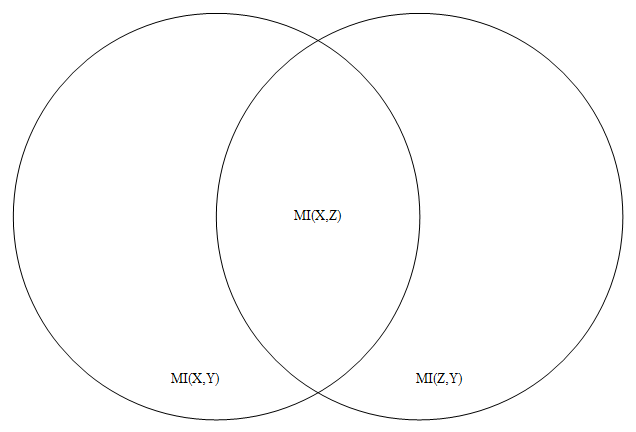# Data Processing Inequality

The Data Processing Inequality is a nice, intuitive inequality about Mutual Information.  Suppose X,Y, Z are random variables and Z is independent of X given Y, then

MI(X,Z) <= MI(X,Y).

See http://www.scholarpedia.org/article/Mutual_information which has an easy one line proof.

We can apply this inequality to a stacked restricted Boltzmann machine (a type of deep neural net).

Let X be a random binary vector consisting of the states of neurons in the first layer.

Let Y be a random binary vector consisting of the states of neurons in the second layer.

And let Z be a random binary vector consisting of the states of neurons in the third layer.

Then

MI(X,Z) <= min( MI(X,Y), MI(Y,Z) ).

Informally, that inequality says that amount of information that can flow from the first layer to the third layer of a stacked RBM deep neural net is less than or equal to the maximum flow rate between the first and second layer.  Also, the amount of information that can flow from the first layer to the third layer is less than or equal to the maximum flow rate between the second and third layer.  This inequality will seem obvious to those who know information theory, but I still think it’s cute.

The above inequality is also sharp in the sense that there are simple examples where the right hand side equals the left hand side.  Consider a Markov Random Field consisting of just three random binary variables X, Y and Z.  Suppose further,  that P(X)=0.5, P(X=Y)=1,  and P(Y=Z)=1.  Then MI(X,Y)=1 bit, MI(Y,Z) =1 bit, and MI(X,Z) =1 bit so both sides of the inequality are 1.

Information theory can also be used to construct a lower bound on the information transfer between the first and third layer.

MI(X,Z) >= MI(Y,X)+MI(Y,Z) – H(Y)

where H(Y) is the entropy of Y (i.e. the information content of the random variable Y).Intuitively, if the sum of the information from X to Y and from Z to Y  exceeds the information capacity of Y, then there must be some information transfer between X and Z.